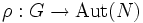# Automorphism group action lemma

## Statement

Suppose$H$ is a group, and$N,G \le H$ are subgroups such that$G \le N_H(N)$. Suppose$\sigma'$ is an automorphism of$H$ such that the restriction of$\sigma'$ to$N$ gives an automorphism$\alpha$ of$N$, and such that$\sigma'$ also restricts to an automorphism of$G$, say$\sigma$. Consider the map:$\rho: G \to \operatorname{Aut}(N)$

that sends an element$g \in G$ to the automorphism of$N$ induced by conjugation by$g$ (note that this is an automorphism since$G \le N_H(N)$). Then, we have:$\rho \circ \sigma = c_\alpha \circ \rho$

where$c_\alpha$ denotes conjugation by$\alpha$ in the group$\operatorname{Aut}(N)$.